Language
Currency
Book: +31(0)20 531 33 32
Monday to Friday 09:00 - 17:301. Select theme (optional)
 Arrival Sat 010203040506070809101112131415161718192021222324252627282930 - Nov, 2019Dec, 2019Jan, 2020Feb, 2020Mar, 2020Apr, 2020May, 2020Jun, 2020Jul, 2020Aug, 2020Sep, 2020Oct, 2020 Nov 23 Number of nights: 12345678910111213141516171819202122232425262728293031 Departure: Sunday 24 Nov 2019 Check availability

## Location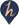## Surroundings

Hotel De Gelderse Poort is close to the village Ooij, near the city Nijmegen. There are various museums and attractions in this area. You can, for example, visit the 'Afrikamuseum' in Berg en Dal, or the 'Museumpark Orientalis', which is part of the national monument 'Heilig Landstichting'. Those who enjoy a good walk or bike ride can indulge in their passion in the lovely nature area 'De Gelderse Poort'. In the hotel's surroundings there are also various golf courses.

# Fletcher Hotel de Gelderse Poort, Ooij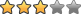Koningin Julianalaan 32, 6576 AS, Ooij

## Book a room at Fletcher Hotel de Gelderse Poort Book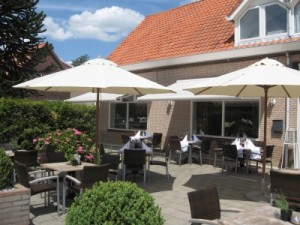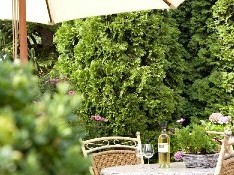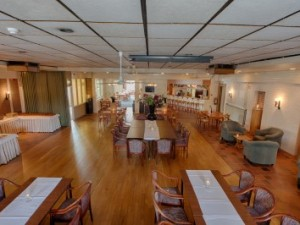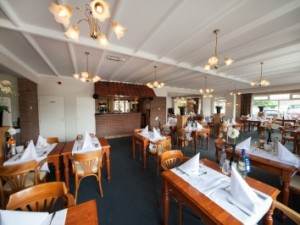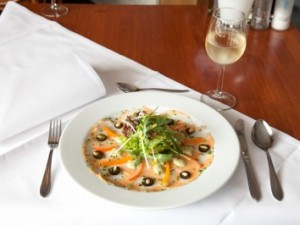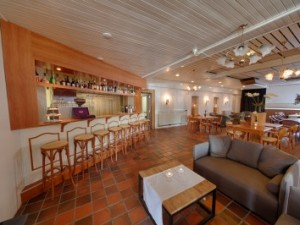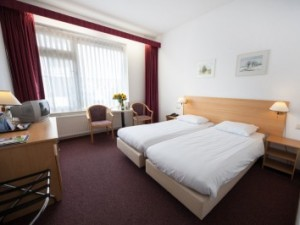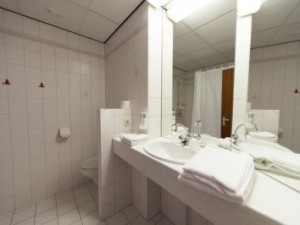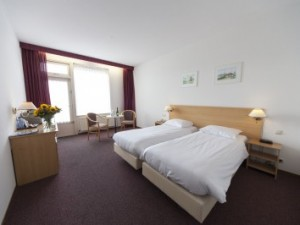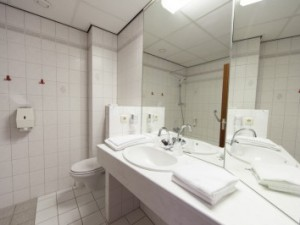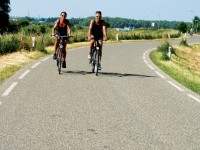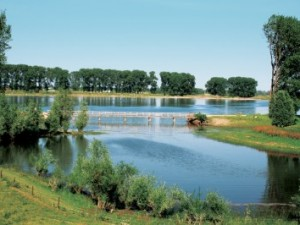Just outside of Nijmegen, in the delightful village of Ooij, is Hotel-Restaurant De Gelderse Poort situated. This charming and comfortable hotel is the perfect place to start your discovery of the Gelderse Poort.

The Gelderse Poort is a unique nature reserve between Arnhem, Nijmegen and Emmerich where you can walk or cycle for hours through unspoilt and varied surroundings. For example, along the Waal river, the famous Ooij polder and the Millingerwaard, all beautiful areas where you can relax. And your enjoyment of the outdoors begins in the morning when you are woken by the sound of a rooster!

Hotel-Restaurant De Gelderse Poort in Ooij, near Nijmegen, is the ideal location for a relaxing weekend or midweek. Be sure to take a look at the page of Package deals for last-minute offers. Then you can quickly get away from the daily routine. Breakfast: Included.
Hotel Rooms: 43
Check-in time: 15:00
Check-out time: 11:00
Hotel Facilities: Elevator, Pets allowed, Restaurant, Terrace, Bar, Meeting Facilities, Non Smoking rooms available, Parking, Internet.
Surcharges and taxes:

• Including 9.00% vat per room per stay
• Excluding eur2.00 city tax per person per night

Arrival
Nights
Departure
Book
 Selected Available On request Not available No arrival day No departure day No arrival and departure day

•

The comfortable double rooms with shower are tastefully decorated and equipped with a direct dial telephone, television, comfortable beds and a bathroom with shower and toilet.

• Sat
23 Nov
• Sun
24 Nov
• Mon
25 Nov
• Tue
26 Nov
• Wed
27 Nov
• Thu
28 Nov
• Fri
29 Nov
• Sat
30 Nov
• Sun
1 Dec
• Mon
2 Dec
• Tue
3 Dec
• Wed
4 Dec
• Thu
5 Dec
• Fri
6 Dec
• Sat
7 Dec
• Sun
8 Dec
• Mon
9 Dec
• Tue
10 Dec
• Wed
11 Dec
• Thu
12 Dec
Standard Rate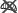2 Maximum nr of persons: 2
•
x
•
x
•
x
•
x
•
x
•
x
•
x
•
x
•
x
•
x
•
x
•
x
•
x
•
x
•
x
•
x
•
x
•
x
•
x
•
x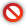Standard Rate Breakfast Included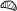2 Maximum nr of persons: 2
•
x
•
x
•
x
•
x
•
x
•
x
•
x
•
x
•
x
•
x
•
x
•
x
•
x
•
x
•
x
•
x
•
x
•
x
•
x
•
xNon Refundable Rate2 Maximum nr of persons: 2
•
x
•
x
•
x
•
x
•
x
•
x
•
x
•
x
•
x
•
x
•
x
•
x
•
x
•
x
•
x
•
x
•
x
•
x
•
x
•
xNon Refundable Rate Breakfast Included2 Maximum nr of persons: 2
•
x
•
x
•
x
•
x
•
x
•
x
•
x
•
x
•
x
•
x
•
x
•
x
•
x
•
x
•
x
•
x
•
x
•
x
•
x
•
x•

The comfortable double rooms with bath are tastefully and comfortably furnished and equipped with direct telephone, WiFi, TV, radio, beds and a bathroom with bath and toilet.

• Sat
23 Nov
• Sun
24 Nov
• Mon
25 Nov
• Tue
26 Nov
• Wed
27 Nov
• Thu
28 Nov
• Fri
29 Nov
• Sat
30 Nov
• Sun
1 Dec
• Mon
2 Dec
• Tue
3 Dec
• Wed
4 Dec
• Thu
5 Dec
• Fri
6 Dec
• Sat
7 Dec
• Sun
8 Dec
• Mon
9 Dec
• Tue
10 Dec
• Wed
11 Dec
• Thu
12 Dec
Internet Rate2 Maximum nr of persons: 2
•
x
•
x
•
x
•
x
•
x
•
x
•
x
•
x
•
x
•
x
•
x
•
x
•
x
•
x
•
x
•
x
•
x
•
x
•
x
•
xStandard Rate2 Maximum nr of persons: 2
•
x
•
x
•
x
•
x
•
x
•
x
•
x
•
x
•
x
•
x
•
x
•
x
•
x
•
x
•
x
•
x
•
x
•
x
•
x
•
xStandard Rate Breakfast Included2 Maximum nr of persons: 2
•
x
•
x
•
x
•
x
•
x
•
x
•
x
•
x
•
x
•
x
•
x
•
x
•
x
•
x
•
x
•
x
•
x
•
x
•
x
•
xNon Refundable Rate2 Maximum nr of persons: 2
•
x
•
x
•
x
•
x
•
x
•
x
•
x
•
x
•
x
•
x
•
x
•
x
•
x
•
x
•
x
•
x
•
x
•
x
•
x
•
xNon Refundable Rate Breakfast Included2 Maximum nr of persons: 2
•
x
•
x
•
x
•
x
•
x
•
x
•
x
•
x
•
x
•
x
•
x
•
x
•
x
•
x
•
x
•
x
•
x
•
x
•
x
•
x• Sat
23 Nov
• Sun
24 Nov
• Mon
25 Nov
• Tue
26 Nov
• Wed
27 Nov
• Thu
28 Nov
• Fri
29 Nov
• Sat
30 Nov
• Sun
1 Dec
• Mon
2 Dec
• Tue
3 Dec
• Wed
4 Dec
• Thu
5 Dec
• Fri
6 Dec
• Sat
7 Dec
• Sun
8 Dec
• Mon
9 Dec
• Tue
10 Dec
• Wed
11 Dec
• Thu
12 Dec
Standard Rate2 Maximum nr of persons: 2
•
x
•
x
•
x
•
x
•
x
•
x
•
x
•
x
•
x
•
x
•
x
•
x
•
x
•
x
•
x
•
x
•
x
•
x
•
x
•
xStandard Rate Breakfast Included2 Maximum nr of persons: 2
•
x
•
x
•
x
•
x
•
x
•
x
•
x
•
x
•
x
•
x
•
x
•
x
•
x
•
x
•
x
•
x
•
x
•
x
•
x
•
xNon Refundable Rate2 Maximum nr of persons: 2
•
x
•
x
•
x
•
x
•
x
•
x
•
x
•
x
•
x
•
x
•
x
•
x
•
x
•
x
•
x
•
x
•
x
•
x
•
x
•
xNon Refundable Rate Breakfast Included2 Maximum nr of persons: 2
•
x
•
x
•
x
•
x
•
x
•
x
•
x
•
x
•
x
•
x
•
x
•
x
•
x
•
x
•
x
•
x
•
x
•
x
•
x
•
x• Sat
23 Nov
• Sun
24 Nov
• Mon
25 Nov
• Tue
26 Nov
• Wed
27 Nov
• Thu
28 Nov
• Fri
29 Nov
• Sat
30 Nov
• Sun
1 Dec
• Mon
2 Dec
• Tue
3 Dec
• Wed
4 Dec
• Thu
5 Dec
• Fri
6 Dec
• Sat
7 Dec
• Sun
8 Dec
• Mon
9 Dec
• Tue
10 Dec
• Wed
11 Dec
• Thu
12 Dec
Standard Rate3 Maximum nr of persons: 3
•
x
•
x
•
x
•
x
•
x
•
x
•
x
•
x
•
x
•
x
•
x
•
x
•
x
•
x
•
x
•
x
•
x
•
x
•
x
•
xStandard Rate Breakfast Included3 Maximum nr of persons: 3
•
x
•
x
•
x
•
x
•
x
•
x
•
x
•
x
•
x
•
x
•
x
•
x
•
x
•
x
•
x
•
x
•
x
•
x
•
x
•
xNon Refundable Rate3 Maximum nr of persons: 3
•
x
•
x
•
x
•
x
•
x
•
x
•
x
•
x
•
x
•
x
•
x
•
x
•
x
•
x
•
x
•
x
•
x
•
x
•
x
•
xNon Refundable Rate Breakfast Included3 Maximum nr of persons: 3
•
x
•
x
•
x
•
x
•
x
•
x
•
x
•
x
•
x
•
x
•
x
•
x
•
x
•
x
•
x
•
x
•
x
•
x
•
x
•
x• Sat
23 Nov
• Sun
24 Nov
• Mon
25 Nov
• Tue
26 Nov
• Wed
27 Nov
• Thu
28 Nov
• Fri
29 Nov
• Sat
30 Nov
• Sun
1 Dec
• Mon
2 Dec
• Tue
3 Dec
• Wed
4 Dec
• Thu
5 Dec
• Fri
6 Dec
• Sat
7 Dec
• Sun
8 Dec
• Mon
9 Dec
• Tue
10 Dec
• Wed
11 Dec
• Thu
12 Dec
Standard Rate4 Maximum nr of persons: 4
•
x
•
x
•
x
•
x
•
x
•
x
•
x
•
x
•
x
•
x
•
x
•
x
•
x
•
x
•
x
•
x
•
x
•
x
•
x
•
xStandard Rate Breakfast Included4 Maximum nr of persons: 4
•
x
•
x
•
x
•
x
•
x
•
x
•
x
•
x
•
x
•
x
•
x
•
x
•
x
•
x
•
x
•
x
•
x
•
x
•
x
•
xNon Refundable Rate4 Maximum nr of persons: 4
•
x
•
x
•
x
•
x
•
x
•
x
•
x
•
x
•
x
•
x
•
x
•
x
•
x
•
x
•
x
•
x
•
x
•
x
•
x
•
xNon Refundable Rate Breakfast Included4 Maximum nr of persons: 4
•
x
•
x
•
x
•
x
•
x
•
x
•
x
•
x
•
x
•
x
•
x
•
x
•
x
•
x
•
x
•
x
•
x
•
x
•
x
•
xThis hotel is also suitable for guests with a handicap. The entire hotel is threshold-free and there is a large lift. The rooms are also threshold-free and all have spacious bathrooms with a raised toilet and a shower with anti-slip tiles. Wireless internet is also available.
The hotel facilities are:
* Cosy restaurants.
* A lounge.
* Lift.
* Terrace.
* Roof terrace with a nice view.
* Own parking space.

## Cancellation- and additional conditions Fletcher Hotel de Gelderse Poort

For all our reservations the Uniform Conditions for the Hotel and Catering Industry apply. A different cancellation policy may apply for group bookings. The hotel will contact you within 24 hrs. if this is the case.## Now searching hotels and prices